# NI Circuit Design Community Blog

cancel
Showing results for
Did you mean:13567 Views

## Fuse Model with Thermal Derating in Multisim

Introduction

A fuse is a temperature sensitive device that plays a critical role in circuit protection. Since the operating temperature has an effect on fuse performance and lifetime, it is extremely valuable to be able to model fuse thermal derating in a SPICE environment while the operating temperature should be taken into consideration when selecting the fuse current rating.

This new model implements a very fast acting fuse that includes its thermal derating curve. Simulation will show how the operating current and temperature have an effect on the fuse’s performance and lifetime.

Fuse Model with Thermal Derating Parameters

The Multisim model is developed based on the approach introduced in this paper and is shown in Fig. 1. The implemented fuse model is for the 0603SFV050F/32-2 very fast-Acting chip fuses by TE Connectivity. The parameters used inside the models are as follows:

• the rated current: irs=0.5A
• the nominal cold DCR: RFref=0.860Ω
• the nominal I2t: I2tref=0.0093A2sec

The model requires the fuse resistance at the end of the pre-arcing time, rfm, which is usually provided in the datasheet. This resistance can be determined through laboratory measurements. Another variant to determine the rfm is to consider that the heating process of the fuse is rapid and adiabatic. Knowing the fuse material, the fuse resistance at room temperature, RF, using equation (1), rfm can be calculated considering that the resistance versus temperature is linear: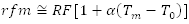where α is the temperature coefficient of the fuse material, Tm is the melting temperature of the fusing material, and T0 is the reference temperature. The length and section area of the fuse are considered constant.

The thermal derating curve is implemented using a lookup table, while the values above were taken from the fuse manufacturer datasheet. The variation of the fuse resistance with temperature and also the variation of the I2t in percent with temperature are all taken into account inside the model.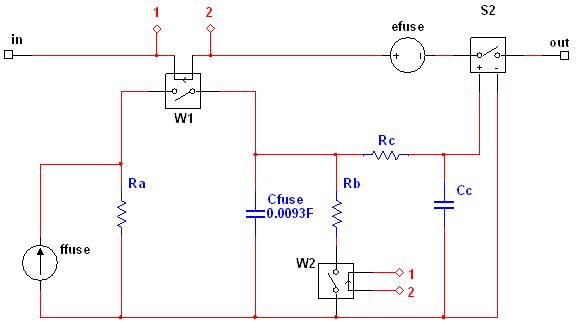Figure 1. Fuse SPICE model internal structure

Note that in the model above the W2 current controlled switch is used for removing the path for Cfuse discharge after the fuse is blown. To view the model of the fuse double click on it and then click on the Edit Model button under the Value tab.

Running the Fuse Model

The fuse is placed in a simple test circuit (attached to the article) to verify its operation in an interactive simulation setup.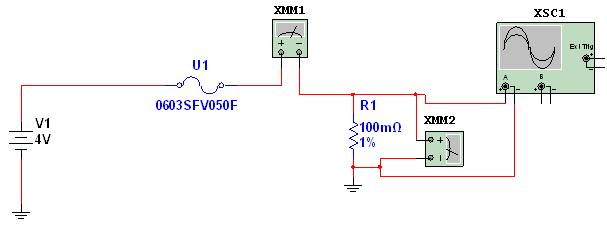Figure 2. Test circuit setup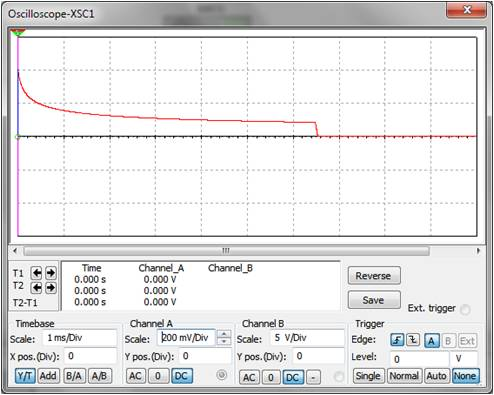Figure 3. Load voltage during when over current passes through the fuse

The Parameter Sweep Analyses can be used, to show the fuse’s response at different currents. In the Analysis Sweep field, the Transient Analysis option must be chosen. Both the Transient and Parameter Sweep Analyses configurations are shown below in Figure 4.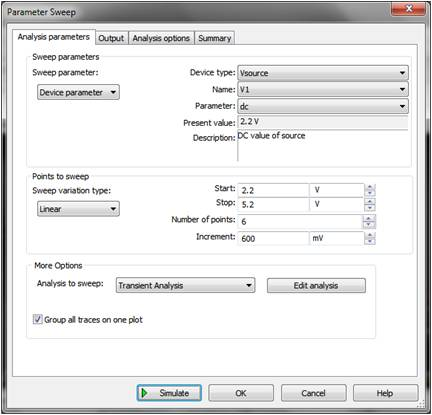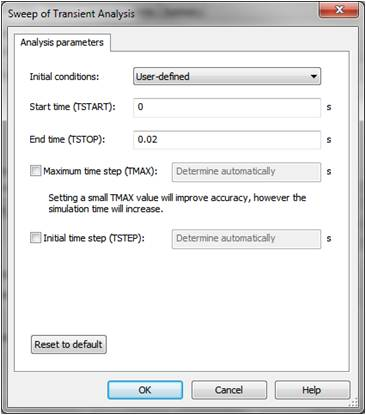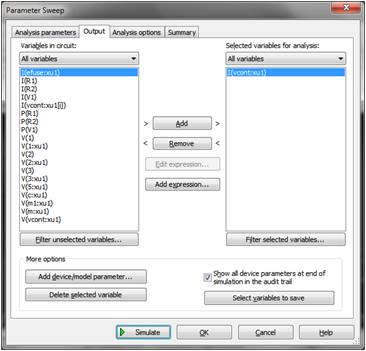Figure 4. Analysis setup in Multisim

Running this sweep analysis, the transient response of the fast acting sweep can be evaluated under different current conditions. This is valuable in determining how fast the fuse will melt and how much energy is needed to do so. The results are shown in Figure 5.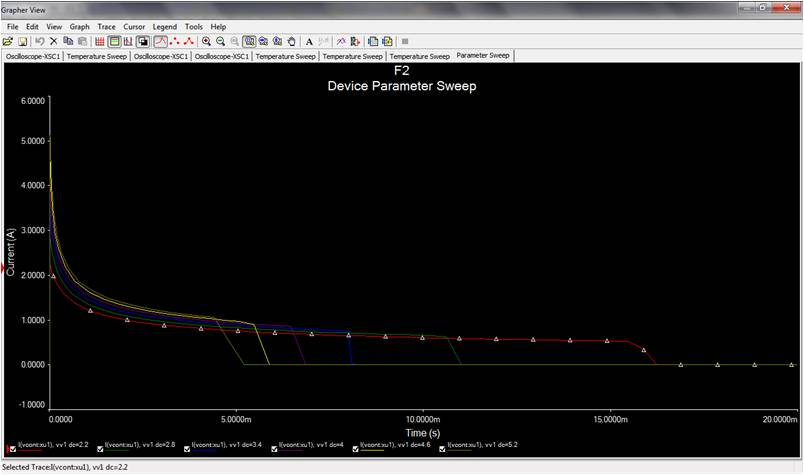Figure 5. Analysis results in Multisim

Moreover, the effect of the ambient temperature could be analyzed in Multisim. It is important to understand how differently the fuse will behave at 75 degrees from a normal 25 degrees room temperature. For this purpose a temperature sweep from 25 to 125 degrees is setup in Multisim to run the same circuit under a constant load current.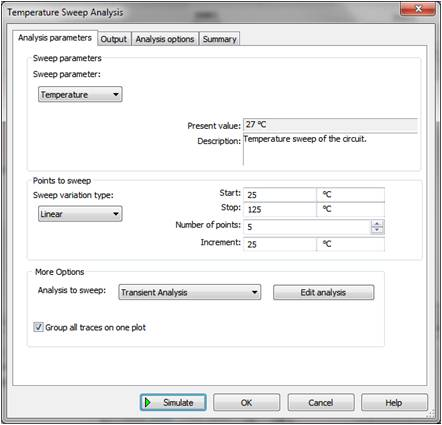Figure 6. Temperature sweep setup in Multisim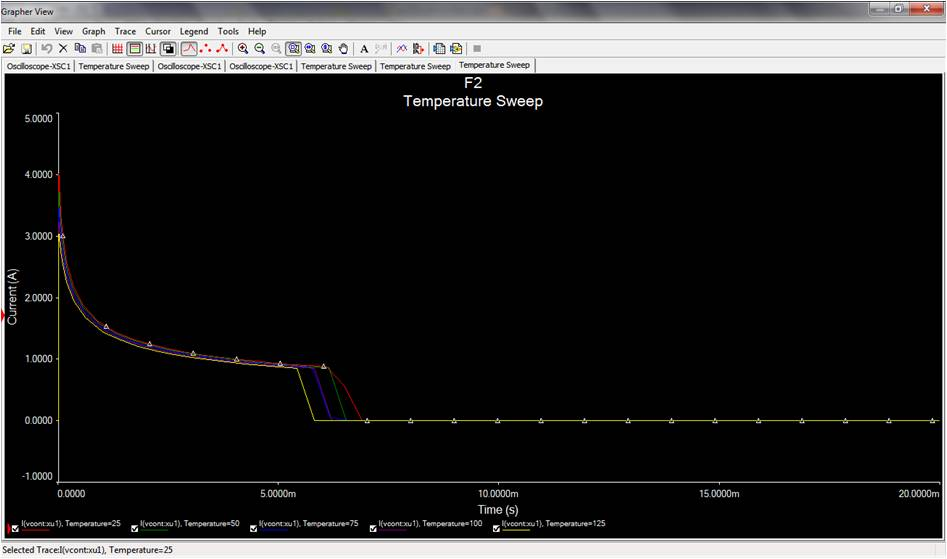Figure 7. Simulation results of the temperature sweep

The ismulation results show that the higher the environment temperature is the faster the switch will respond to the over-current.